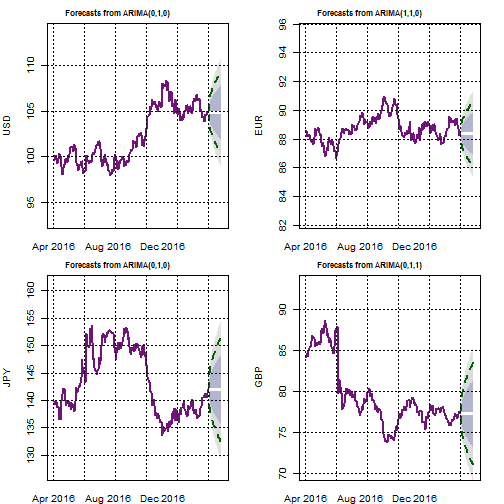# Category Archives: FX

## Trade Weighted Currency Indices Stretch Map

Tue Aug 22 05:23:24 2017

The following report aims to provide a gauge to the current strenght of major currencies. For doing so I use the Bank of England Trade weighted Exchange rate indices and a standardised statistical measures of price deviation to provide an estimate of how stretched major currencies are on a trade weighted perspective.I first calculate the T-stat of the mean price deviations over a rolling period of 61 days. The charts below show the results for each currency over the last 500 days. The purple line represents the median value since 1990-01-03 and the red lines represent the 95% confidence intervals. Therefore if the value is above or below those the deviation of the given currency would be deemed as atypical relative to what #would be expected under a normal distribution and therefore overbought/oversold.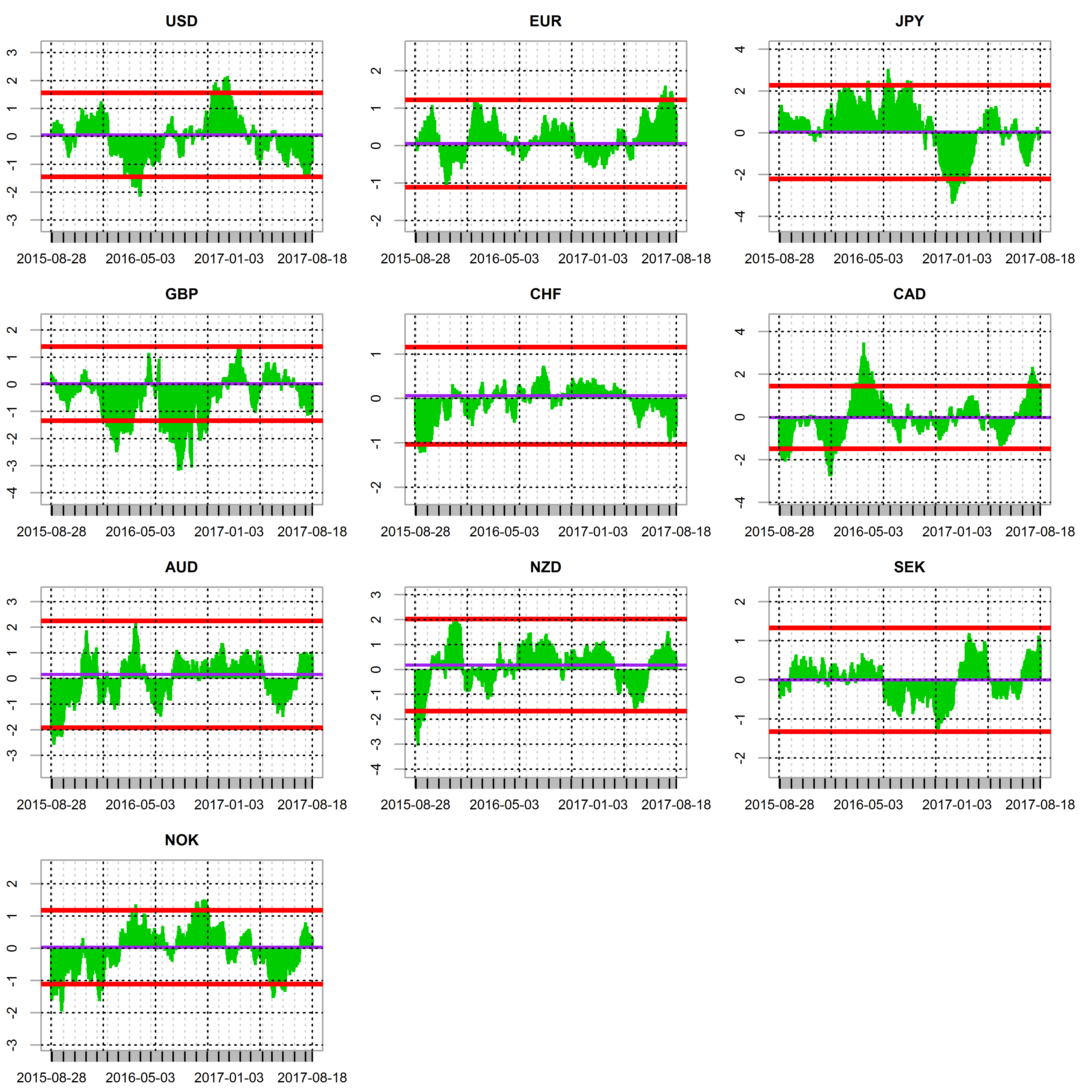The following Map chart shows how stretched the currencies are over time horizons ranging from 1-month to 1-year. The bigger the square the most significant the upside (green) or downside (red) of currencies over the given period.The charts below show how the daily changes in the Trade weighted indices have correlated since January 1990 and since the begining of 2015.Finally, the following provide an ARIMA forecast for each of the trade weighted indices. My script selects the best ARIMA fit over the previous 250-day to generate a forecast for the next 21 days.
It also shows the forecast confidence intervals.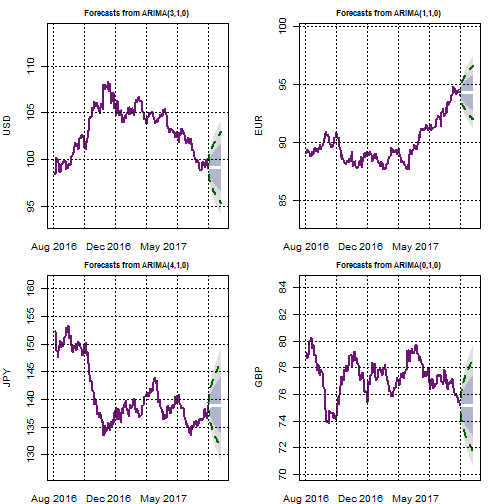## Weekly Changes in G10 FX Trade Weighted Indices

I always liked boxplots. I think they provide a great and very visual way to position current data relative to their history whilst highlighting outliers. This is particularly useful as it helps to put recent moves in context of their past opportunities and possibly highly reversals and/or opportunities. To illustrate this I wrote a quick script in R to grab the BOE G10 Trade weighted indices from the website of the bank of England and posititon the most recent one week move relative to its history of weekly move going back to 1990.
The blue dots represent the most recent observations, the orange dots are the outliers over the period 1990 to date. The boxes emcompasses the observations that fall between the 25% and 75% quantiles. The Blue lines in the box are the median value over the sample and the “wiskers” represent an interval of close to 95%.## Trade Weighted Currency Indices Stretch Map

Tue Jul 04 20:51:00 2017

The following report aims to provide a gauge to the current strenght of major currencies. For doing so I use the Bank of England Trade weighted Exchange rate indices and a standardised statistical measures of price deviation to provide an estimate of how stretched major currencies are on a trade weighted perspective.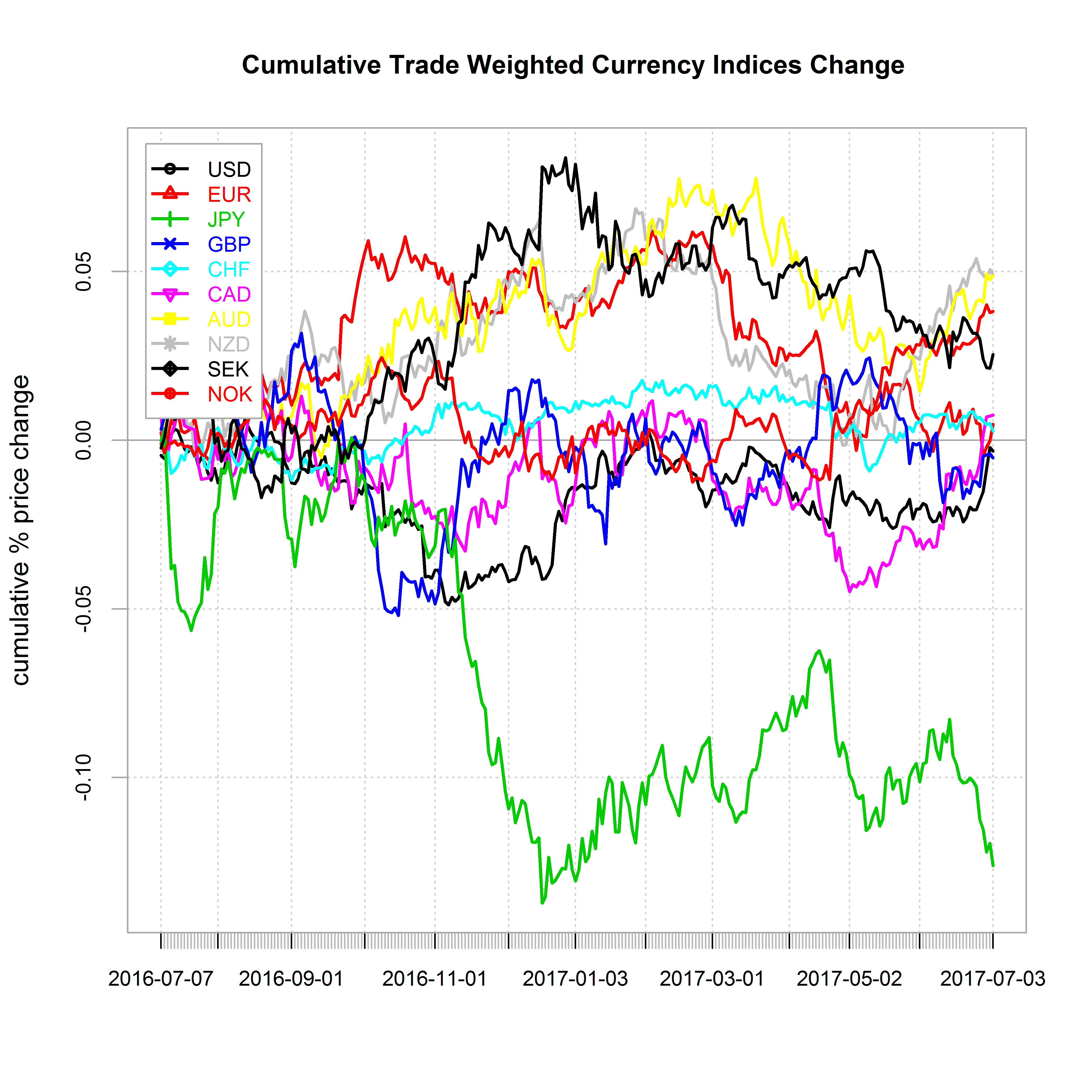I first calculate the T-stat of the mean price deviations over a rolling period of 61 days. The charts below show the results for each currency over the last 500 days. The purple line represents the median value since 1990-01-03 and the red lines represent the 95% confidence intervals. Therefore if the value is above or below those the deviation of the given currency would be deemed as atypical relative to what #would be expected under a normal distribution and therefore overbought/oversold.The following Map chart shows how stretched the currencies are over time horizons ranging from 1-month to 1-year. The bigger the square the most significant the upside (green) or downside (red) of currencies over the given period.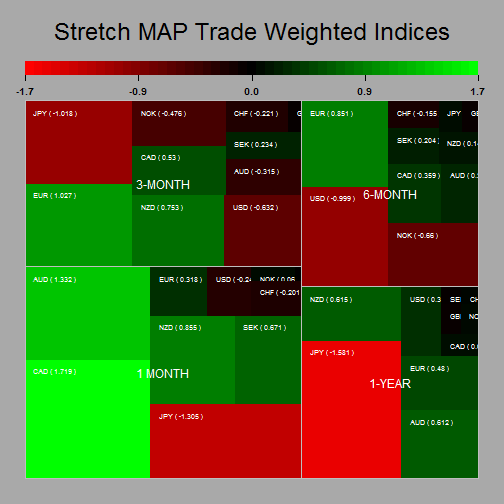The charts below show how the daily changes in the Trade weighted indices have correlated since January 1990 and since the begining of 2015.Finally, the following provide an ARIMA forecast for each of the trade weighted indices. My script selects the best ARIMA fit over the previous 250-day to generate a forecast for the next 21 days.
It also shows the forecast confidence intervals.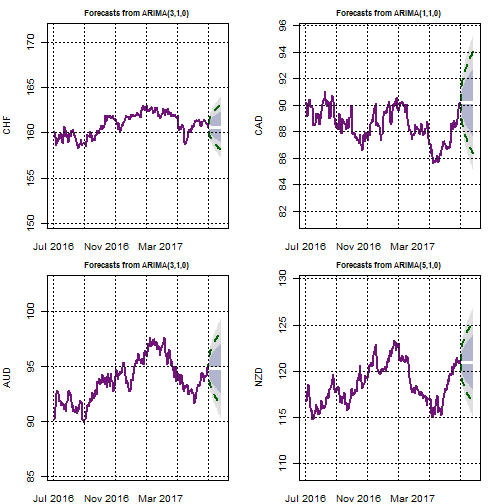## G10 FX Implied Volatilities: Cheap or Expensive ?

The following report provides a granular analysis of implied volatilities within G10 FX. I use primarily the same formatting than for my G10FX positioning report to estimate how extended the 1-month FX implied volatilities are over various time horizon.

The first set of charts shows the historical T-stat of the 1-day changes in 1-month implied volatilities over a rolling period of 61-days. This is my statistical metric to quantify how stretched the implied volatilities are, but clearly other time period could be used as shown further down on in that report. The purple line represents the median value since 1996 and the red lines represent the 95% confidence intervals. Therefore if the value is above or below those the deviation of the given implied volatility should be deemed as atypical relative to what would be expected under a normal distribution (I am not saying that implied volatilities have a normal behaviour to be clear….) and therefore overbought/oversold.The below charts shows the current implied volatilities relative to their historical distributions since 1996. Once again the red lines delimit the 95% confidence intervals and the purple line the median value. The blue line indicates the most current level of 1-month implied volatility.Finally the below shows a stretch map of the T-Stats to help visualise how much implied volatilities have departed from their equilibrium levels over time horizons ranging from 1-month to 6-month. The bigger the square the most significant the observed upside (Green) or downside (Red) of the implied volatility over the given period.## GBPUSD Implied Volatility Level and Steepness of Volatility Curve

I recently have read a paper titled The yen/dollar exchange rate in 1998: views from options markets written by the Bank of England back in November 1998. This got me to think of how I could best represent in one chart the relationship between the slope of the implied volatility term structure of and the nominal level o the 1-month volatility. In the following example I have applied this to the GBPUSD implied volatility as I thought this would be interesting to look at in the light of the forthcomint BREXIT negotiations and tonight elections….

Anyhow to do this, I regress the 1, 3, 6 and 12-month GBPUSD implied volatilies against their time values for the period 1996 to Mayr 2017 (i.e 5513 volatility curves). I derived the volatility curve slopes t_stats for each day and then classified the 1-month volatilities into three groups as a function of the significance level of the slope t-stats. The chart below shows the 1-month implied volatiliy over the full period. When the volatility curve slope was positvely significant at 95% critical threshold the data is shown in green, When there was a signicantly negative slope at the 95% critical threshold the data is shown in red and pale blue for the remainder. I think this is a neat way highlight that time of high volatility are associated with a volatility curve that slope downwardly and vice-versa.Below are the

## Trade Weighted Currency Indices Stretch Map

Mon May 29 07:01:07 2017

The following report aims to provide a gauge to the current strenght of major currencies. For doing so I use the Bank of England Trade weighted Exchange rate indices and a standardised statistical measures of price deviation to provide an estimate of how stretched major currencies are on a trade weighted perspective.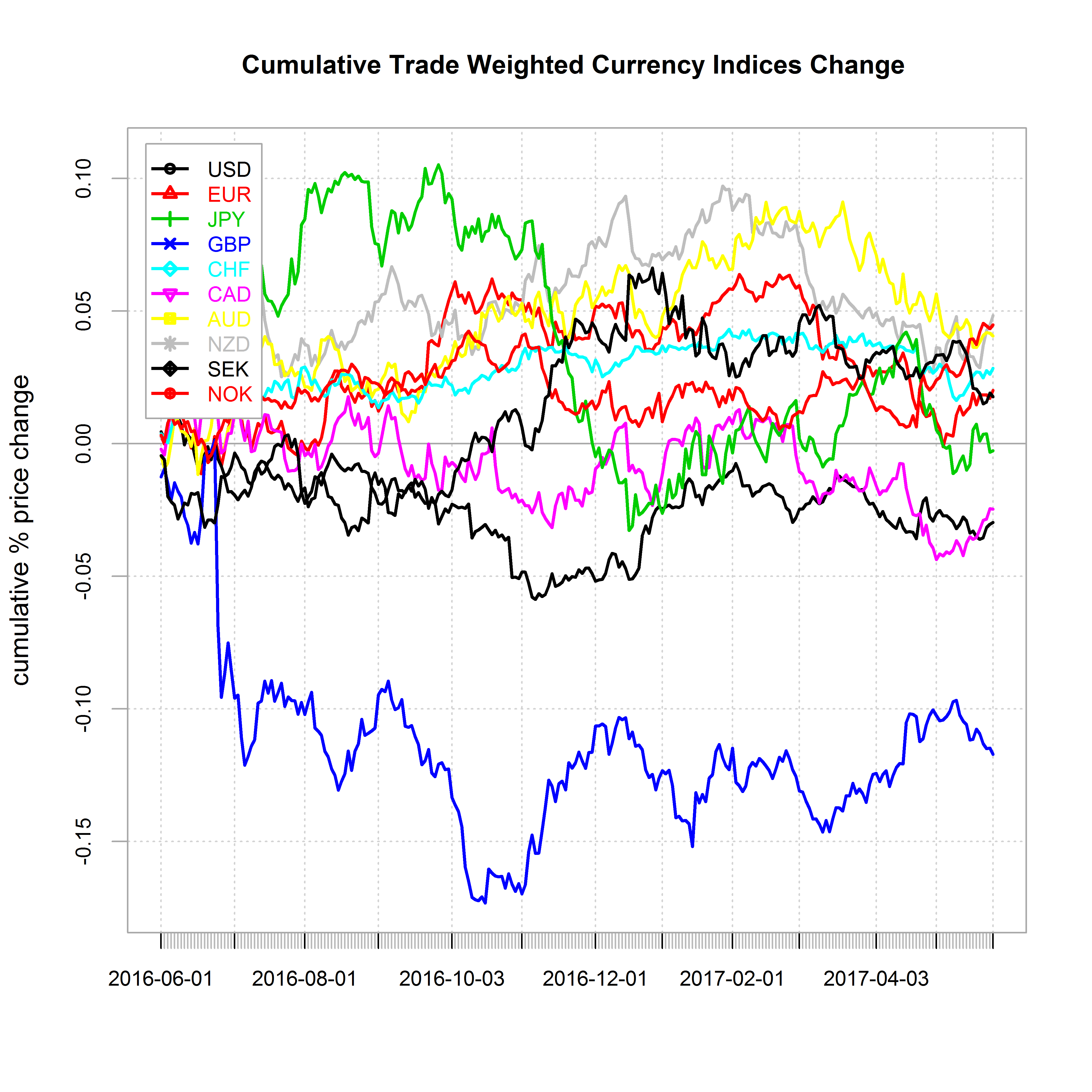I first calculate the T-stat of the mean price deviations over a rolling period of 61 days. The charts below show the results for each currency over the last 500 days. The purple line represents the median value since 1990-01-03 and the red lines represent the 95% confidence intervals. Therefore if the value is above or below those the deviation of the given currency would be deemed as atypical relative to what #would be expected under a normal distribution and therefore overbought/oversold.The following Map chart shows how stretched the currencies are over time horizons ranging from 1-month to 1-year. The bigger the square the most significant the upside (green) or downside (red) of currencies over the given period.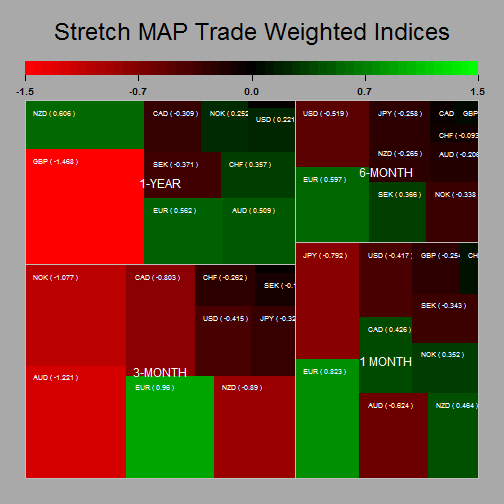The charts below show how the daily changes in the Trade weighted indices have correlated since January 1990 and since the begining of 2015.Finally, the following provide an ARIMA forecast for each of the trade weighted indices. My script selects the best ARIMA fit over the previous 250-day to generate a forecast for the next 21 days.
It also shows the forecast confidence intervals.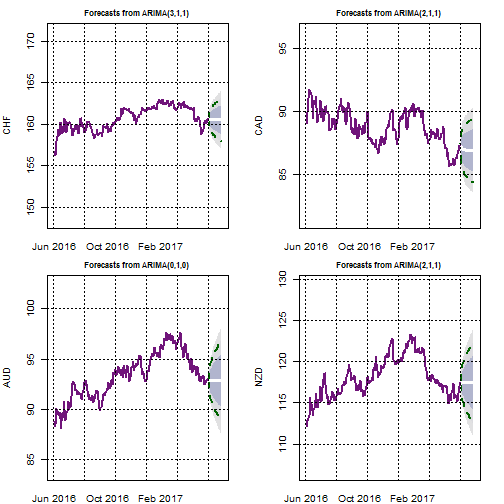## G10 FX Implied Volatilities: Cheap or Expensive ?

The following report provides a granular analysis of implied volatilities within G10 FX. I use primarily the same formatting than for my G10FX positioning report to estimate how extended the 1-month FX implied volatilities are over various time horizon.

The first set of charts shows the historical T-stat of the 1-day changes in 1-month implied volatilities over a rolling period of 61-days. This is my statistical metric to quantify how stretched the implied volatilities are, but clearly other time period could be used as shown further down on in that report. The purple line represents the median value since 1996 and the red lines represent the 95% confidence intervals. Therefore if the value is above or below those the deviation of the given implied volatility should be deemed as atypical relative to what would be expected under a normal distribution (I am not saying that implied volatilities have a normal behaviour to be clear….) and therefore overbought/oversold.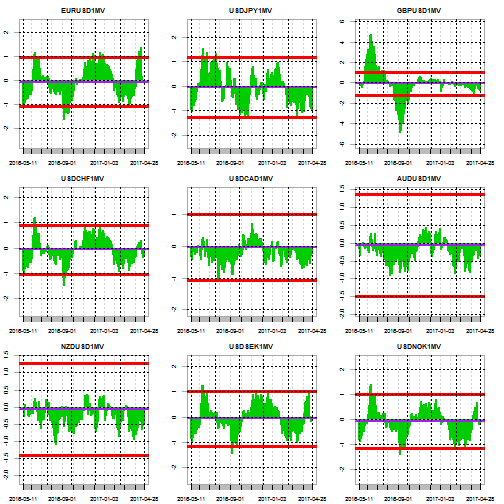The below charts shows the current implied volatilities relative to their historical distributions since 1996. Once again the red lines delimit the 95% confidence intervals and the purple line the median value. The blue line indicates the most current level of 1-month implied volatility.Finally the below shows a stretch map of the T-Stats to help visualise how much implied volatilities have departed from their equilibrium levels over time horizons ranging from 1-month to 6-month. The bigger the square the most significant the observed upside (Green) or downside (Red) of the implied volatility over the given period.## GBPUSD Implied Volatility Level and Steepness of Volatility Curve

I recently have read a paper titled The yen/dollar exchange rate in 1998: views from options markets written by the Bank of England back in November 1998. This got me to think of how I could best represent in one chart the relationship between the slope of the implied volatility term structure of and the nominal level o the 1-month volatility. In the following example I have applied this to the GBPUSD implied volatility as I thought this would be interesting to look at in the light of the forthcomint BREXIT negocaitions….

Anyhow to do this, I regress the 1, 3, 6 and 12-month GBPUSD implied volatilies against their time values for the period 1996 to Mayr 2017 (i.e 5513 volatility curves). I derived the volatility curve slopes t_stats for each day and then classified the 1-month volatilities into three groups as a function of the significance level of the slope t-stats. The chart below shows the 1-month implied volatiliy over the full period. When the volatility curve slope was positvely significant at 95% critical threshold the data is shown in green, When there was a signicantly negative slope at the 95% critical threshold the data is shown in red and pale blue for the remainder. I think this is a neat way highlight that time of high volatility are associated with a volatility curve that slope downwardly and vice-versa.Below are the

## Trade Weighted Currency Indices Stretch Map

Wed Apr 26 20:34:07 2017

The following report aims to provide a gauge to the current strenght of major currencies. For doing so I use the Bank of England Trade weighted Exchange rate indices and a standardised statistical measures of price deviation to provide an estimate of how stretched major currencies are on a trade weighted perspective.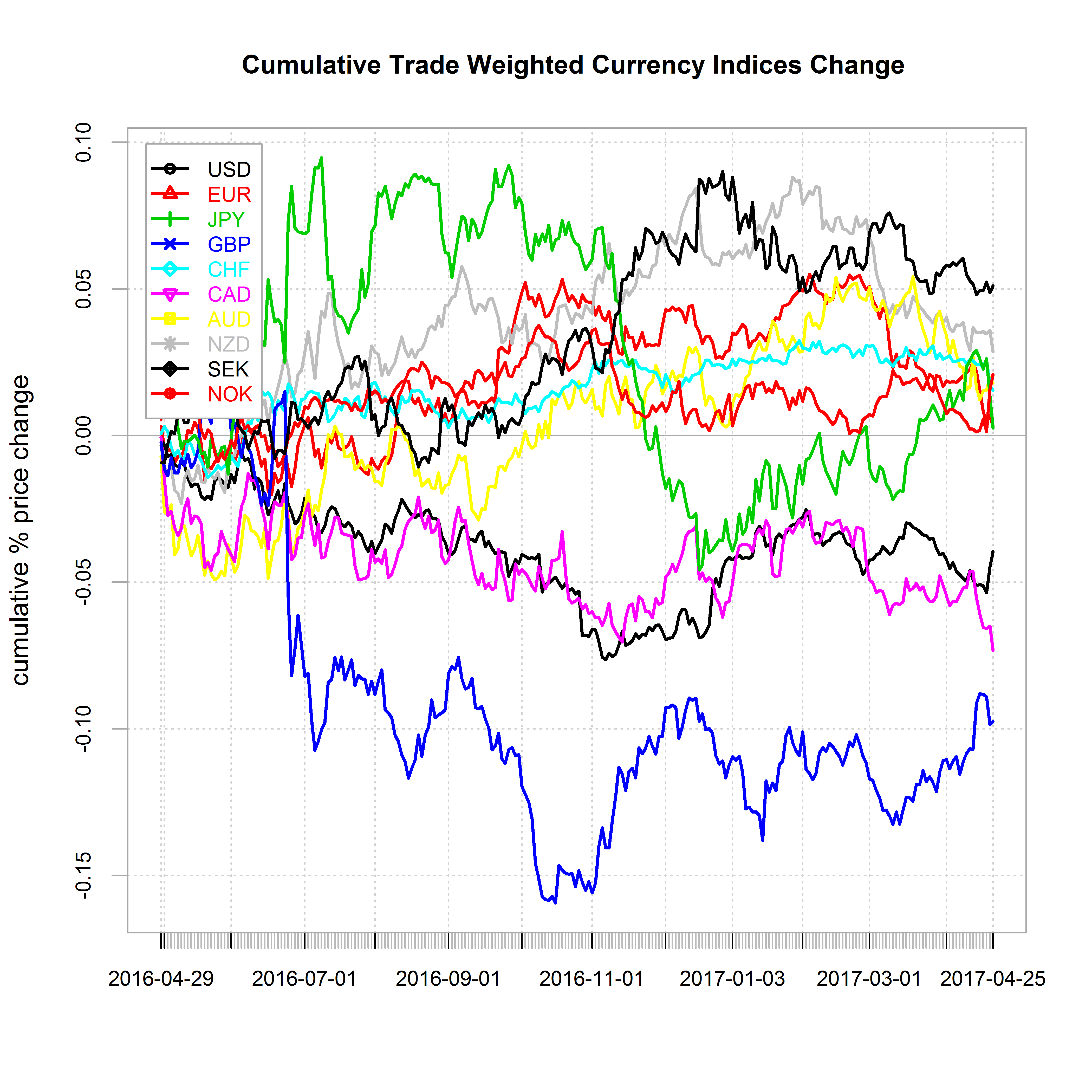I first calculate the T-stat of the mean price deviations over a rolling period of 61 days. The charts below show the results for each currency over the last 500 days. The purple line represents the median value since 1990-01-03 and the red lines represent the 95% confidence intervals. Therefore if the value is above or below those the deviation of the given currency would be deemed as atypical relative to what #would be expected under a normal distribution and therefore overbought/oversold.The following Map chart shows how stretched the currencies are over time horizons ranging from 1-month to 1-year. The bigger the square the most significant the upside (green) or downside (red) of currencies over the given period.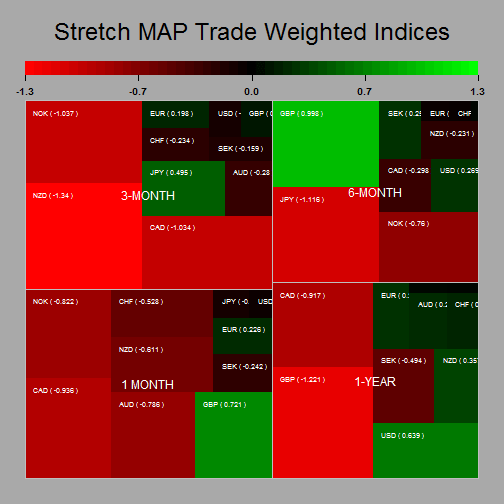The charts below show how the daily changes in the Trade weighted indices have correlated since January 1990 and since the begining of 2015.Finally, the following provide an ARIMA forecast for each of the trade weighted indices. My script selects the best ARIMA fit over the previous 250-day to generate a forecast for the next 21 days.
It also shows the forecast confidence intervals.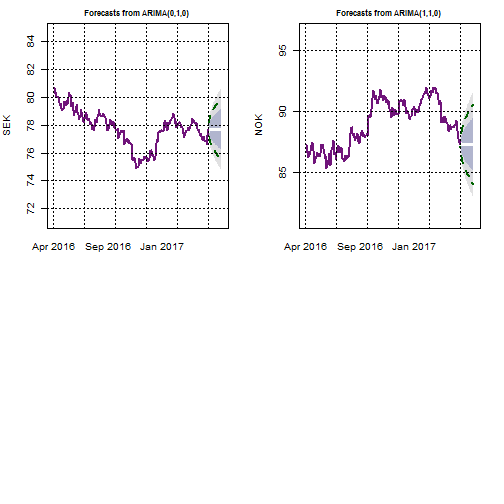## Trade Weighted Currency Indices Stretch Map

Wed Apr 05 19:45:03 2017

The following report aims to provide a gauge to the current strenght of major currencies. For doing so I use the Bank of England Trade weighted Exchange rate indices and a standardised statistical measures of price deviation to provide an estimate of how stretched major currencies are on a trade weighted perspective.I first calculate the T-stat of the mean price deviations over a rolling period of 61 days. The charts below show the results for each currency over the last 500 days. The purple line represents the median value since 1990-01-03 and the red lines represent the 95% confidence intervals. Therefore if the value is above or below those the deviation of the given currency would be deemed as atypical relative to what #would be expected under a normal distribution and therefore overbought/oversold.The following Map chart shows how stretched the currencies are over time horizons ranging from 1-month to 1-year. The bigger the square the most significant the upside (green) or downside (red) of currencies over the given period.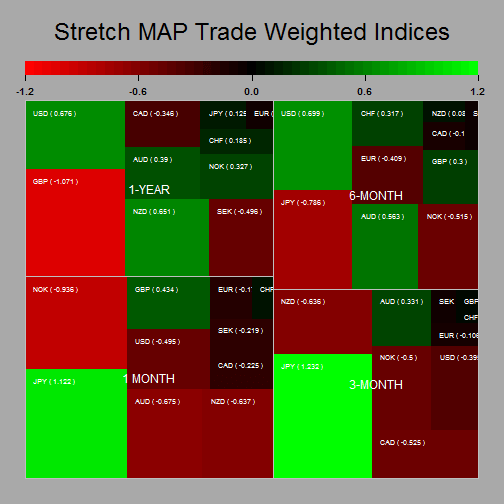The charts below show how the daily changes in the Trade weighted indices have correlated since January 1990 and since the begining of 2015.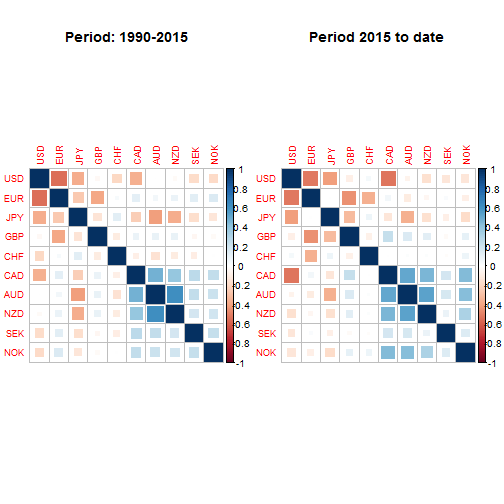Finally, the following provide an ARIMA forecast for each of the trade weighted indices. My script selects the best ARIMA fit over the previous 250-day to generate a forecast for the next 21 days.
It also shows the forecast confidence intervals.